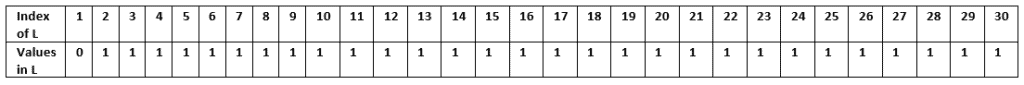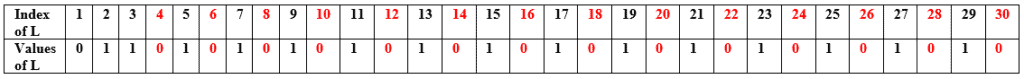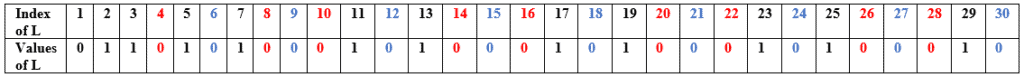## 1. Overview

In this tutorial, we’ll discuss the Sieve of Eratosthenes algorithm. We’ll also present the time complexity analysis of the algorithm.

## 2. Theoretic Idea

Given a number, we need to find all the prime numbers up to the input integer.

The Sieve of Eratosthenes algorithm is simple, but one of the most efficient ways to find them in a segment. The first step of the algorithm is to write down all the numbers fromto the input number.

For any given input number greater than, the first prime would always be. So we’ll create a list of consecutive integers fromto a specified input integer. At this point, we found the first prime number. Next, we’ll remove all the integers from our list, which are the multiples of(sinceis the first prime number).

We’ll continue choosing the next prime numbers and remove the multiples of the current prime number from our list until the current prime number is less than or equal to. Finally, all the integers which will remain in our list are our prime numbers.

## 3. Pseudocode

We discussed the steps of the Sieve of Eratosthenes algorithm, let’s see the pseudocode now:Here we take a numberas an input and create an arrayof size. The index of the arrayrepresents the numbers, and the values inside the arraydenotes whether it’s a prime number or not. If the value insideis, its a composite number. Similarly, if the value insideis, it’s a prime number.

We know thatis not a prime number, so we start the algorithm by initializingtoand the rest of the indices to. In each iteration, we set a loop value and check whether it’s a composite or prime number. If it’s a prime number, we remove all the integers from our list which are the multiples of that prime number by assigning them.

We’ll run the algorithm until the current loop value is less than or equal to. Finally, we return all the indices whose value is.

## 4. Example

Let’s take. The first step is to create a listof sizeand assign the first index toand the rest with:Now we’ll start the iteration. Initially, we set loop value toand check the index of the arrayif it contains the valueor not. If the indexincontains, we assignto all the indices, which are the multiples of current loop value:Next, we’ll set the value of the loop to, and continue the same process:The next loop value would be, but as we can see, its value is. So we’ll go to the next index that’sand repeat the same process:Our next prime would be, but according to the algorithm, we’ll stop when the current prime number is less than or equal to. Hereis, thereforeis. So we’ll not continue with the next prime number and terminate the algorithm. The algorithm returns the indices which have value, and these are all prime numbers:## 5. Time Complexity

The core part of this algorithm is to mark the composite numbers and remove them from the listby assigning. Now to mark a composite number and assign the valueto it takestime. The real complexity of this algorithm lies in the number of times the loops run to mark the composite numbers.

In order to remove the composite numbers, we assign the valueto the multiples of the current prime number in. Now let’s assume our current prime number is. In the first iteration, we’ll markelements. Like this, when our current prime number is, we assigntocomposite numbers. The total number times we runs the loop would be equal to:Let’s solve this equation:Using Harmonic Progression of the sum of primes, it can be proved that:Therefore, total time complexity of the Sieve of Eratosthenes algorithm would be.

## 6. Conclusion

In this tutorial, we’ve discussed the Sieve of Eratosthenes algorithm in detail. We presented the pseudocode of the algorithm and analyzed the time complexity.